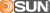2022-2023 Yavapai College Catalog
 Select a Catalog 2023-2024 Yavapai College Catalog 2022-2023 Yavapai College Catalog [PREVIOUS CATALOG YEAR] 2021-2022 Yavapai College Catalog [PREVIOUS CATALOG YEAR] 2020-2021 Catalog [PREVIOUS CATALOG YEAR] 2019-20 Catalog [PREVIOUS CATALOG YEAR] 2018-19 Catalog [PREVIOUS CATALOG YEAR]
Nov 29, 2023
 HELP 2022-2023 Yavapai College Catalog [PREVIOUS CATALOG YEAR] Print-Friendly Page (opens a new window)

# MAT 152 - College AlgebraMAT 1151.
Description: Modeling of applications using linear, quadratic, exponential and logarithmic functions. Introduction to solving systems of equations using matrices. Note: Computer use and graphing calculator required (TI-83/84 recommended). Duplicate credit for MAT 152 and MAT 182 will not be awarded.

Prerequisites: MAT 097  or a satisfactory score on the mathematics skills assessment.

General Education Competency: Quantitative Literacy

Credits: 3
Lecture: 3
Course Content:
1. Linear Functions
2. Quadratic and other nonlinear functions
3. Exponential and logarithmic functions
4. Polynomial functions
5. Systems of equations and matrices
6. Technology in mathematics

Learning Outcomes:
1. Recognize trends in data. (1-4,6)
2. Create suitable functions that model data using technology. (1-4,6)
3. Analyze an application using a function developed from data. (1-4,6)
4. Add, subtract and multiply matrices in the context of an application. (5,6)
5. Solve a system of equations using matrices and technology. (5,6)
6. Solve polynomial, exponential, logarithmic, rational, and power equation algebraically. (1-4)
7. Solve polynomial, exponential, logarithmic, rational, and power equation graphically.  (1-4)
8. Predict function values from polynomial, exponential, logarithmic, rational, and power models. (1-4)